# Excel DAX - Editing a Calculated Field

You can edit a calculated field to modify it. But, before you edit a calculated field, you should know where it is stored. That means, in which table the calculated field is stored. This holds both for implicit and explicit calculated fields. A calculated field can be associated with only one table in a Data Model.

## Finding Calculated Fields

To find the calculated fields in the Data Model, do the following −

• Click the Advanced tab on the Ribbon in the Power Pivot window.
• Click the Show Implicit Calculated Fields.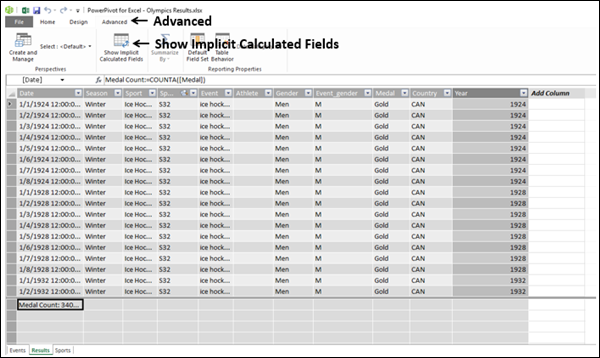• Click the Diagram View.As you can see in the above screenshot, Show Implicit Calculated Fields is highlighted on the Ribbon. If it is not highlighted, click it again.

You can also observe that there are 4 checkboxes – Columns, Calculated Fields, Hierarchies, and KPIs. By default, all the 4 are selected.

• Uncheck the boxes - Columns, Hierarchies and KPIs.

This will leave only Calculated Fields box checked.As seen in the above screenshot, only the Results table has fields displayed. The other two tables are blank. This shows that only the Results table has calculated fields. You can also observe that the implicit calculated fields have an icon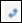displayed, whereas the explicit calculated field – Medal Count does not have that icon.

## Viewing Calculated Fields in the Table

You can view the calculated fields in the Table as follows −

• Click the calculated field.
• Right-click and select Go To in the dropdown list.The table will appear in Data View.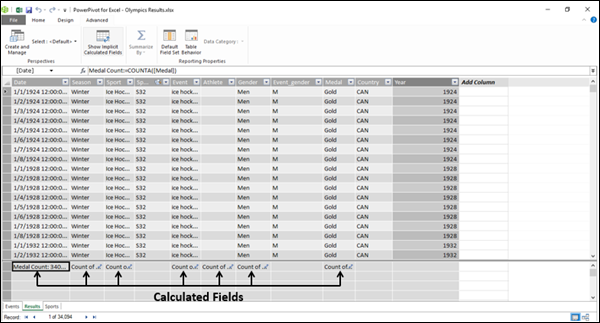As seen in the above screenshot, the calculated fields appear in the calculation area of the table.

## Changing a Calculated Field in the Table

You can change the formula used for a calculated field in the table.

• Click the calculated field in the table in data view of the Data Model.
• Select the formula in the formula bar – to the right side of :=.

The formula will get highlighted.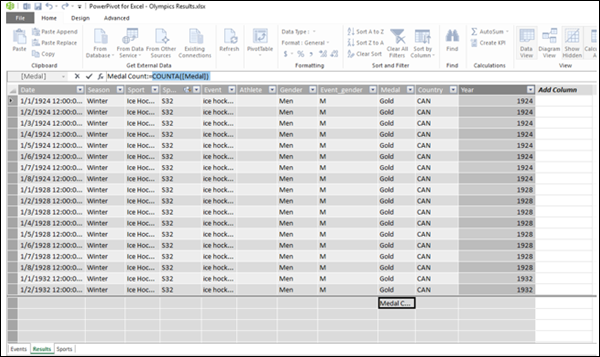• Type the new formula.
• Press Enter.

## Renaming a Calculated Field in the Data Model

You can change the name of a calculated field in the Data Table either in Data View or Diagram View.

### Renaming a Calculated Field in the Data View

• Click the calculated field in the table in data view of the Data Model.
• Select the calculated field name in the formula bar – to the left side of :=.

The calculated field name will get highlighted.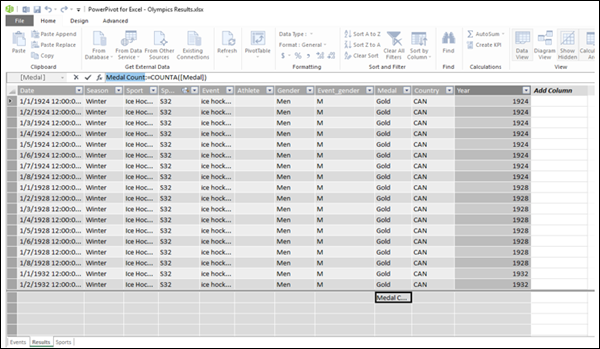• Type the new name for the calculated field.
• Press Enter.

### Renaming a Calculated Field in the Diagram View

• Right-click the calculated field name in the table in the diagram view.
• Click Rename in the dropdown list.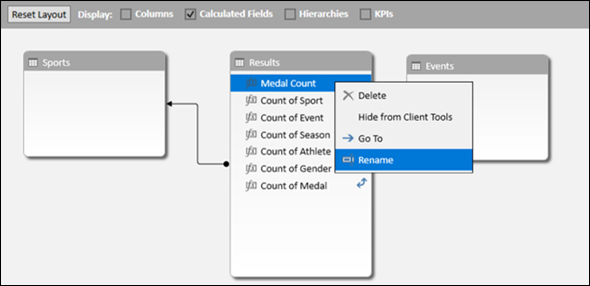The name will get into editing mode. Type the new name for the calculated field.

## Viewing Calculated Fields in the Excel Window

You can view the calculated fields in the Excel window as follows −

• Click the POWERPIVOT tab on the Ribbon.
• Click Calculated Fields in the Calculations group.
• Click Manage Calculated Fields in the dropdown list.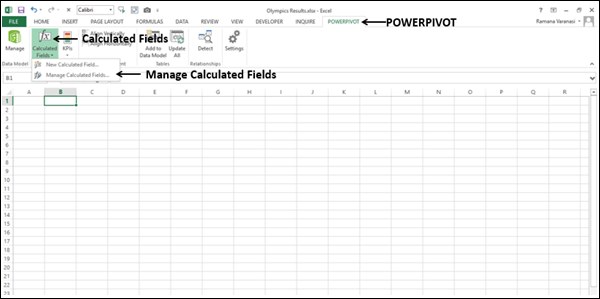Manage Calculated Fields dialog box appears. The names of the explicit calculated fields in the Data Model appear in the dialog box.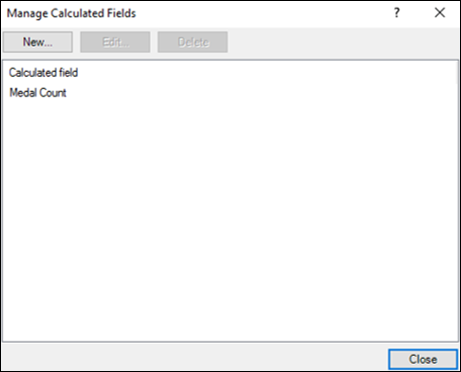## Changing a Calculated Field in the Manage Calculated Fields

You can change a calculated field in the Manage Calculated Fields dialog box.

• Click the Medal Count.
• Click the Edit button.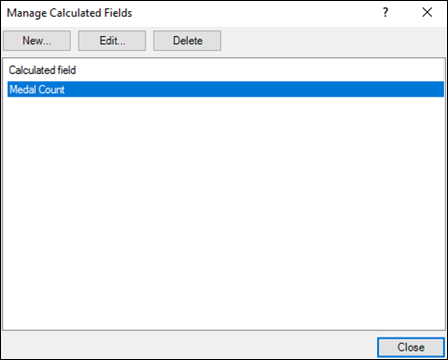Calculated Field dialog box appears.

• Select the formula to the right of = in the formula box.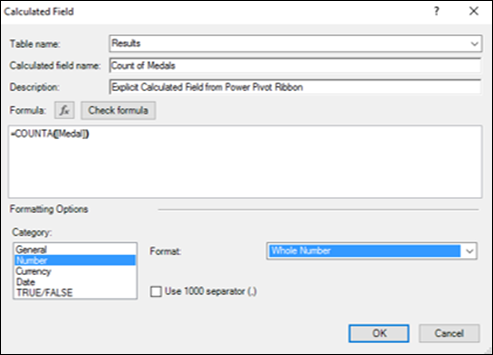• Type the new Formula.
• Click OK.
• Click Close in the Manage Calculated Fields dialog box.

## Renaming a Calculated Field in the Manage Calculated Fields

You can rename a calculated field in the Manage Calculated Fields dialog box.

• Click the Medal Count.
• Click the Edit button.

Calculated Field dialog box appears.

• Select the name in the calculated field name box.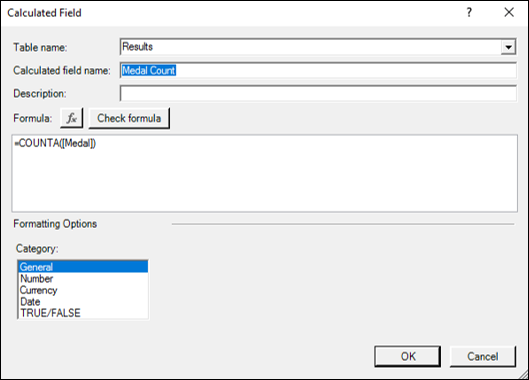• Type the new name for the calculated field.
• Click OK.
• Click Close in the Manage Calculated Fields dialog box.

## Moving a Calculated Field in the Data Model

You can move a calculated field within the calculation area of the table in which it is created. But, it cannot be moved to another table.

• Right-click the calculated field.
• Click Cut.
• Move the pointer to a different place in the calculation area of the same table.
• Click Paste.

Note − It does not really matter where the calculated field is within the calculation area of the table because the data references in the DAX formula of the calculated field are by the column names and are stated explicitly.